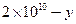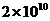Chapter 34, Problem 15PE

Chapter
Section
Textbook Problem

(a) What Hubble constant corresponds to an approximate age of the universe of 1010 y? To get an approximate value, assume the expansion rate is constant and calculate the speed at which two galaxies must move apart to be separated by 1 Mly (present average galactic separation) in a time of 1010 y. (b) Similarly, what Hubble constant corresponding to a universe approximatelyold?

To determine

(a)

The Hubble constant corresponding to an approximate age of the universe1010 years.

Explanation

Given:

Age of Universe =1010 years

Separation between galaxies = 1 Mly

Formula used:

Find velocity using:

Velocity = distance/time

Then find H0which is equal tovelocity/time

Calculation:

Velocity = distance/timev = (1 Mly/1010Y)= (1 x 106ly1010y) x ( 9.46 x1012Km1ly) x (1y3

To determine

(b)

The Hubble constant corresponding to a universe approximatelyy old.

Still sussing out bartleby?

Check out a sample textbook solution.

See a sample solution

The Solution to Your Study Problems

Bartleby provides explanations to thousands of textbook problems written by our experts, many with advanced degrees!

Get Started

Find more solutions based on key concepts